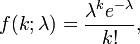# Propability of hitchhikers being picked up

SimpliciusH
I apologize if I'm posting this in the wrong subforum, I wasn't quite sure where statistics and distribution questions should go. I have major problems understanding the concepts involved with this kind of stuff.

## Homework Statement

On a country road an average of 30 cars pass per hour. Four hitch-hikers are standing near a sign and waiting for a lift. Supposing each driver is glad to take exactly one hitch-hiker, what is the probability that all the hitch-hikers will have found someone to take them to their destination after 10 minutes?

## Homework Equations

Poisson distribution## The Attempt at a Solution

I'm not quite sure how to approach this. At first glance this seems to be a Poisson distribution since I'm searching for the probability of 4 cars passing by in 10 minutes, and any particular car passing by doesn't seem to (in my opinion) change the probability of any other car passing by.So I took k=4, since I was interested in four cars passing by and I took lambda as 5, I got a probability of 0.17546 (17.5%), which intuitively seems a bit low considering lambda.

Is this naive approach correct or am I missing something?

Thanks for the help!

Edit:
A second after posting I realized that I've only calculated the probability for exactly four cars passing by, but the set conditions are also fulfilled if any number of cars greater than 4 pass by in those 10 minutes. How can I account for this?

Homework Helper
Gold Member
Use P(X ≥ 4) = 1 - P(X < 4)

SimpliciusH
Use P(X ≥ 4) = 1 - P(X < 4)

Ok, that seems blindingly obvious in retrospect, have no idea how I missed it.
Thank you for the help! :)

This is then the correct answer:
P(X ≥ 4) = 1 - P(X < 4) = 1 - P(X = 0) - P(X=1) - P(X=2) - P(X=3)
P(X ≥ 4) = 0,73497

A probability of 73,5%.

Homework Helper
Gold Member
Ok, that seems blindingly obvious in retrospect, have no idea how I missed it.
Thank you for the help! :)

This is then the correct answer:
P(X ≥ 4) = 1 - P(X < 4) = 1 - P(X = 0) - P(X=1) - P(X=2) - P(X=3)
P(X ≥ 4) = 0,73497

A probability of 73,5%.

I wouldn't phrase it as a percent. Probabilities are numbers between 0 and 1.

SimpliciusH
I wouldn't phrase it as a percent. Probabilities are numbers between 0 and 1.

Will remember that, I'm new to this so please don't refrain from pointing out any other things like that.